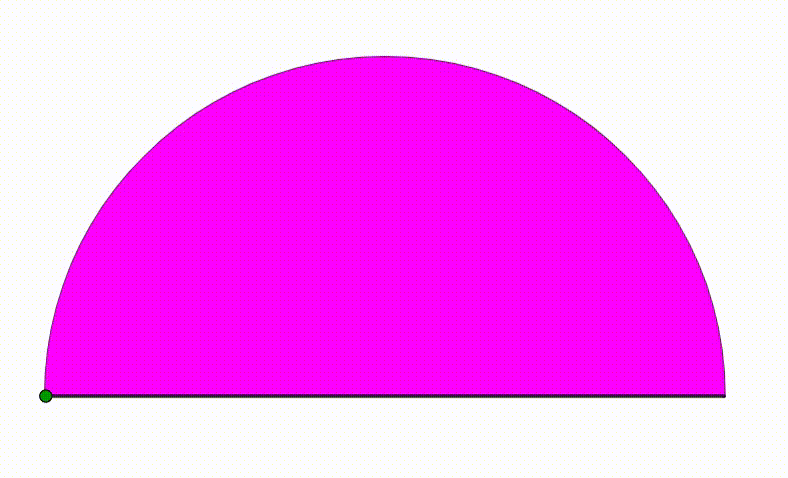# Dynamic Geometry: P32

Geometry Level 5The diagram shows a black semicircle. The pink and yellow semicircles are tangent to each other and internally tangent to the black semicircle. They are growing and shrinking freely so that the sum of their radius is always equal to the black semicircle's radius. We draw a red vertical segment using the common point between the pink and yellow semicircle. At last we inscribed two circles (green and cyan) so they are tangent to the red line, to the black semicircle and to one of the two bottom semicircle. The green and blue point are the intersection points between the red segment and the circles. When the distance between the blue and green point is maximum, the fraction of the white area can be expressed as $\dfrac{a}{b}$ where $a$ and $b$ are coprime positive integers. Find $a+b$.

×